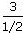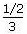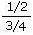Number Magic

Question :
What is a 'Complex Fraction' ?Here, numerator is 3 and the denominator is 1/2.Here, numerator is 1/2 and the denominator is 3.Here, numerator is 1/2 and the denominator is 3/4.LINKSDISCLAIMERCONTACT US